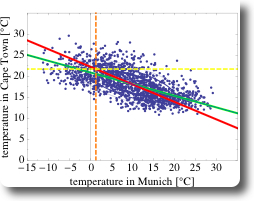# planetwater

ground- water, geo- statistics, environmental- engineering, earth- science

## Regression 102 (Mathematica, Meteorological Data)

The NUPUS conference is over, the first snow of the year has fallen, a good friend is married — now I have finally some time to continue with some examples related to regression and actual weather data. I have promised that since quite a while now. Sorry!

In the first post of this mini-series on regression I looked at some basic properties of traditional regression analysis. Today I will look at two real-world examples of meteorological data and apply some of the methods of the first post. I will use some of the features introduced in Mathematica version 7 for plotting meteorological as well as spatial data. I think you can find a really great introduction at the Mathematica Blog as well as at the mathematica help-site. In the next post, I will look at some disadvantages of traditional regression analysis.

The novel features of mathematica make it fairly easy to look at the daily mean air temperatures in Munich and in Frankfurt (Figure 1). Since the two cities are located fairly close to each other, their daily mean temperatures are fairly similar. The orange dots which indicate Frankfurt are roughly at the same location in the scatter-plot as the black dots which indicate Munich.Figure 1: Daily mean air temperature in Munich and Frankfurt over time

It gets a little bit trickier, if we want to look at a different kind of scatter-plot: not at a time-series as in Figure 1 but at a scatter-plot similar to the heights of the pairs of fathers and sons (Figure 1 in the previous post, for example). Ulises at Wolfram, one of the two authors of the Weather Patterns Blog Post at the Wolfram Blog, was so kind to write a wicked little filter for that purpose, which I am sharing in the mathematica workbooks for this post (see links at the end of the post). This filter involves the Mathematica functions Intersection and Alternatives. As we have seen on Figure 1, at the same date the mean air temperature at both cities is fairly similar, three things can be expected:

• the scatterplot is expected to point upwards
• the point-cloud is expected to be narrowly confined (in contrast to the corresponding figure (Figure 1) in the case of Galton’s fathers- and sons- heights)
• the means for Munich and Frankfurt are expected to be similar

All the expectations are met, as shown on Figure 2. Additionally, the regression line and the SD line are almost identical, which is due to the fact that the correlation coefficient r is very close to 1.Figure 2: Scatter-plot of pairs of daily mean air temperature in Munich and in Frankfurt, Germany

As a second example, let’s compare the daily mean air temperatures in Munich and in Cape Town, South Africa (Figure 3). Since both cities are on different hemispheres, annual cycle of temperatures are phase shifted by half a year. Additionally, the range of encountered temperatures is smaller than in Munich, and always above zero in Cape Town. The corresponding scatter-plot is shown on Figure 4. Due to the phase shift, the correlation is negative and the cloud of the points of the scatter-plot is pointing towards the bottom right of the chart.Figure 3: Daily mean air temperature in Munich and Cape Town over timeFigure 4: Scatter-plot of pairs of daily mean air temperature in Munich and in Cape Town, Germany

What are the differences between the two data-sets using data from Munich-Frankfurt and from Munich-Cape Town?

• if the temperature in Munich is high, then the temperature in Frankfurt is also high (and vice versa), hence there is a positive correlation in temperature in Munich and in Frankfurt: \$\$r = 0.95\$\$.
• if the temperature in Munich is high, then the temperature in Cape Town is low (and vice versa), hence there is a negative correlation in temperature in Munich and in Capetown: \$\$r= -0.66\$\$
• the correlation between Munich and Frankfurt is stronger than between Munich and Cape Town. This is also the reason, why the SD line and the regression line are more similar in the case of Munich and Frankfurt than in the second data-set.

Here are the links to the Mathematica workbooks for this post:

In the next post, I will look at some of the properties of this regression analysis.

Written by Claus

October 23rd, 2009 at 2:12 pm

Posted in

Tagged with ,